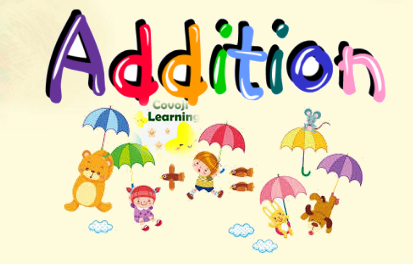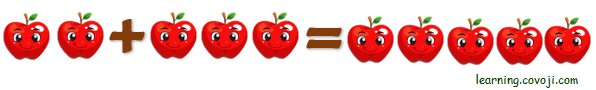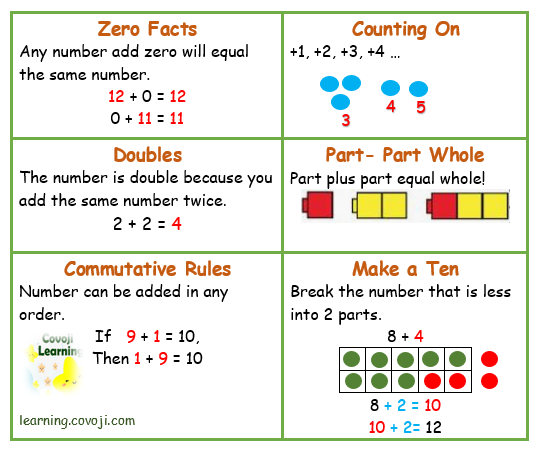Addition is the mathematical operation, calculating the sum of two numbers or quantities. “+” is the symbol of addition. Addition key words are:

• total
• sum
• plus
• combine
• join
• increase
• together